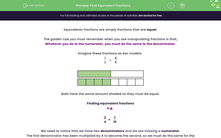# Find Equivalent Fractions

In this worksheet, student will practise simplifying fractions and making equivalent fractions.Key stage:  KS 4

Year:  GCSE

GCSE Subjects:   Maths

GCSE Boards:   Pearson Edexcel, OCR, Eduqas, AQA,

Curriculum topic:   Number, Fractions, Decimals and Percentages

Curriculum subtopic:   Structure and Calculation Fractions

Popular topics:   Equivalent Fractions worksheets

Difficulty level:#### Worksheet Overview

Equivalents fractions are simply fractions that are equal.

The golden rule you must remember when you are manipulating fractions is that;

Whatever you do to the numerator, you must do the same to the denominator.

Imagine these fractions as bar models.

 1 2
=
 4 8Both have the same amount shaded so they must be equal.

Finding equivalent fractions

e.g.

 3 4
=
 ? 16

We need to notice that we have two denominators and we are missing a numerator.

The first denominator has been multiplied by 4 to become the second, so we must do the same for the numerator.

3 x 4 = 12

 3 4
=
 12 16

Simplifying a fraction

To simplify a fraction (make the numbers smaller), we have to find the highest common factor.

This is the largest number we can divide both numbers by.

When we have found this, we need to divide both the numerator and denominator by this amount.

e.g. Simplify:

 45 120

The highest common factor of these two numbers is 5, so we have to divide both numbers by 5:

 45 120
=
 45 ÷ 5 120 ÷ 5
=
 9 20

In this activity, you will find equivalent fractions and simplify fractions into their lowest possible components.

Make sure you have your times tables and knowledge of factors at the ready to support you!

### What is EdPlace?

We're your National Curriculum aligned online education content provider helping each child succeed in English, maths and science from year 1 to GCSE. With an EdPlace account you’ll be able to track and measure progress, helping each child achieve their best. We build confidence and attainment by personalising each child’s learning at a level that suits them.

Get started